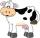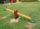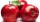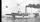# Expression of a variable from the formula - math word problems

#### Number of problems found: 931

• CombinationsIf the number of elements increase by 3, it increases the number of combinations of the second class of these elements 5 times. How many are the elements?
• Two diagonalsThe rhombus has a side length 12 cm and length of one diagonal 21 cm. What is the length of the second diagonal?
• Cuboid - ratiosThe sizes of the edges of the cuboid are in the ratio 2: 3: 5. The smallest wall have area 54 cm2. Calculate the surface area and volume of this cuboid.
• Rectangle diagonalsIt is given rectangle with area 24 cm2 a circumference 20 cm. The length of one side is 2 cm larger than length of second side. Calculate the length of the diagonal. Length and width are yet expressed in natural numbers.
• HypotenuseCalculate the length of the hypotenuse of a right triangle if the length of one leg is 4 cm and its content area is 16 square centimeters.
• MO-Z5-3-66 tilesThe picture shows a square tiles with side 10 dm which is composed of four identical small rectangles and squares. Circumference of small square is five times smaller than the circumference of the entire tile. Determine the dimensions of the rectangle.
• DiagonalsGiven a rhombus ABCD with a diagonalsl length of 8 cm and 12 cm. Calculate the side length and content of the rhombus.
• Chocolate rollThe cube of 5 cm chocolate roll weighs 30 g. How many calories will contain the same chocolate roller of a prism shape with a length of 0.5 m whose cross section is an isosceles trapezoid with bases 25 and 13 cm and legs 10 cm. You know that 100 g of this
• CowsAgricultural cooperative has increased the number of housed cows by 14% to 285 units. By how many cows increased agricultural cooperative the number of cows?
• Cuboid easyThe cuboid has the dimensions a = 12 cm, b = 9 cm, c = 36 cm. Calculate the length of the body diagonal of the cuboid.
• Difference AP 4Calculate the difference of the AP if a1 = 0.5, a2 + a3 = -1.1
• PotatoesDaniela and Michael would jointly dug potatoes for 7.5 hours. But if Daniela was working alone she would take 2.5 hours more as if he were working with Michael. Determine how much for the work done by Michael himself and how much Daniela herself.
• The swingTo swing the two girls. Aneta weight 45 kg and Simon 35 kg weight. How far should sit Simon from the middle of swing so it is balanced, if we know that Aneta is sitting at distance 1,5m? How far are girls sitting apart?
• Apples 5In six crates are 45 kg of apples. In five crates were the same amounth and in one crate was 3 kg of apples more. How many kg of apples were in each crate?
• Four integersFnd four consecutive integers so that the product of the first two is 70 times smaller than the product of the next two.
• MO SK/CZ Z9–I–3John had the ball that rolled into the pool and it swam in the water. Its highest point was 2 cm above the surface. Diameter of circle that marked the water level on the surface of the ball was 8 cm. Determine the diameter of John ball.
• Cruise linerCruise liner runs along the river between points A and B. The journey downstream takes 40 minutes 1 hour upriver. The flow rate of the river is 3 km/h. What is the speed of cruise liner?
• MoonWe see Moon in the perspective angle 28'. Moon's radius is 1740 km at the time of the full moon. Calculate the mean distance of the Moon from the Earth.
• Cuboid surfaceDetermine surface area of cuboid if its volume is 52.8 cm cubic and length of the two edges are 2 cm and 6 cm.
• Cuboid - VabFind the surface of the cuboid when its volume is 52.8 cubic centimeters, and the length of its two edges is 2 centimeters and 6 centimeters.

Do you have an interesting mathematical word problem that you can't solve it? Submit a math problem, and we can try to solve it.

We will send a solution to your e-mail address. Solved examples are also published here. Please enter the e-mail correctly and check whether you don't have a full mailbox.

Please do not submit problems from current active competitions such as Mathematical Olympiad, correspondence seminars etc...